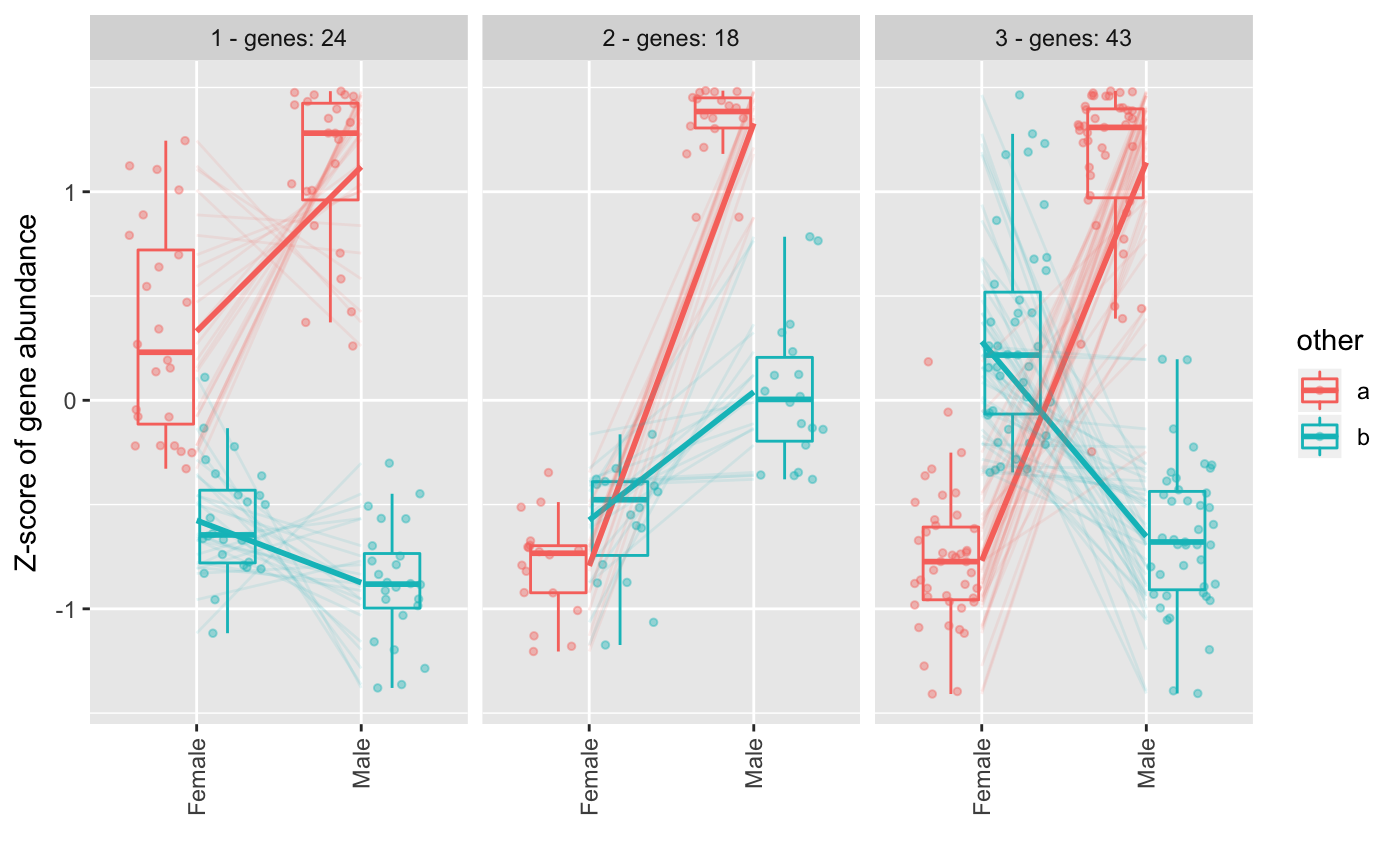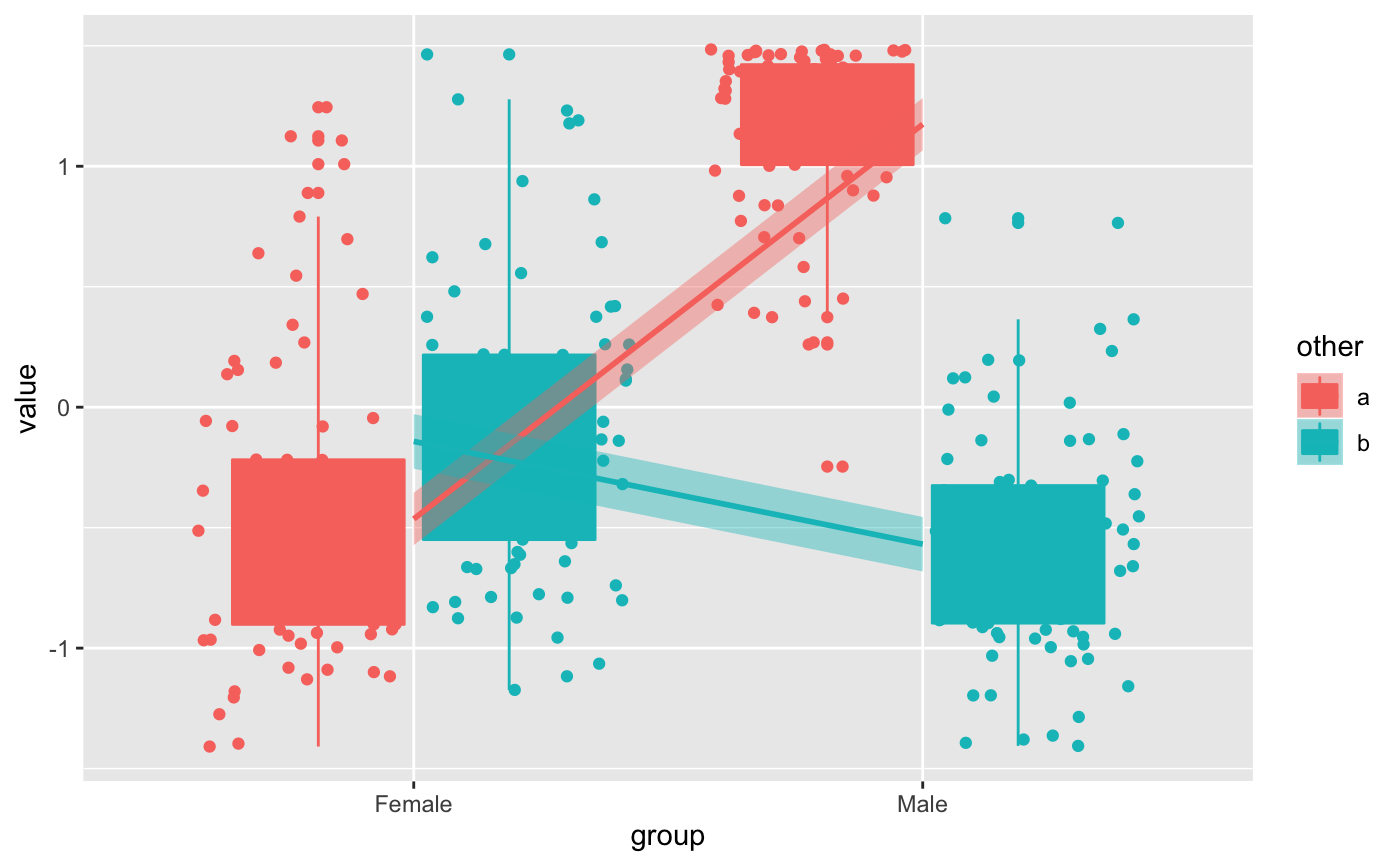Note that this function doesn't calculate significant difference between groups, so the matrix used as input should be already filtered to contain only genes that are significantly different or the most interesting genes to study.

degPatterns(ma, metadata, minc = 15, summarize = "merge",
time = "time", col = NULL, consensusCluster = FALSE,
reduce = FALSE, cutoff = 0.7, scale = TRUE, pattern = NULL,
groupDifference = NULL, eachStep = FALSE, plot = TRUE,
fixy = NULL)

## Arguments

ma log2 normalized count matrix data frame with sample information. Rownames should match ma column names row number should be the same length than p-values vector. integer minimum number of genes in a group that will be return character column name in metadata that will be used to group replicates. If the column doesn't exist it'll merge the time and the col columns, if col doesn't exist it'll use time only. For instance, a merge between summarize and time parameters: control_point0 ... etc character column name in metadata that will be used as variable that changes, normally a time variable. character column name in metadata to separate samples. Normally control/mutant Indicates whether using ConsensusClusterPlus or cluster::diana() boolean remove genes that are outliers of the cluster distribution. boxplot function is used to flag a gene in any group defined by time and col as outlier and it is removed from the cluster. Not used if consensusCluster is TRUE. This is deprecated. boolean scale the ma values by row numeric vector to be used to find patterns like this from the count matrix. As well, it can be a character indicating the genes inside the count matrix to be used as reference. Minimum abundance difference between the maximum value and minimum value for each feature. Please, provide the value in the same range than the ma value ( if ma is in log2, groupDifference should be inside that range). Whether apply groupDifference at each stem over time variable. This only work properly for one group with multiple time points. boolean plot the clusters found vector integers used as ylim in plot

## Value

list wiht two items:

• df is a data.frame with two columns. The first one with genes, the second with the clusters they belong.

• pass is a vector of the clusters that pass the minc cutoff.

• plot ggplot figure.

• hr clustering of the genes in hclust format.

• profile normalized count data used in the plot.

• raw data.frame with gene values summarized by biological replicates and with metadata information attached.

• summarise data.frame with clusters values summarized by group and with the metadata information attached.

• normalized data.frame with the clusters values as used in the plot.

• benchmarking plot showing the different patterns at different values for clustering cuttree function.

• benchmarking_curve plot showing how the numbers of clusters and genes changed at different values for clustering cuttree function.

## Details

It can work with one or more groups with 2 or more several time points. Before calculating the genes similarity among samples, all samples inside the same time point (time parameter) and group (col parameter) are collapsed together, and the mean value is the representation of the group for the gene abundance. Then, all pair-wise gene expression is calculated using cor.test R function using kendall as the statistical method. A distance matrix is created from those values. After that, cluster::diana() is used for the clustering of gene-gene distance matrix and cut the tree using the divisive coefficient of the clustering, giving as well by diana. Alternatively, if consensusCluster is on, it would use ConsensusClusterPlus to cut the tree in stable clusters. Finally, for each group of genes, only the ones that have genes higher than minc parameter will be added to the figure. The y-axis in the figure is the results of applying scale() R function, what is similar to creating a Z-score where values are centered to the mean and scaled to the standard desviation by each gene.

The different patterns can be merged to get similar ones into only one pattern. The expression correlation of the patterns will be used to decide whether some need to be merged or not.

## Examples

data(humanGender)
library(SummarizedExperiment)
library(ggplot2)
ma <- assays(humanGender)[][1:100,]
des <- colData(humanGender)
des[["other"]] <- sample(c("a", "b"), 85, replace = TRUE)
res <- degPatterns(ma, des, time="group", col = "other")#> Working with 100 genes.#> Working with 85 genes after filtering: minc > 15#> Joining, by = "merge"#> Joining, by = "merge"# Use the data yourself for custom figures
ggplot(res[["normalized"]],
aes(group, value, color = other, fill = other)) +
geom_boxplot() +
geom_point(position = position_jitterdodge(dodge.width = 0.9)) +
# change the method to make it smoother
geom_smooth(aes(group=other), method = "lm")